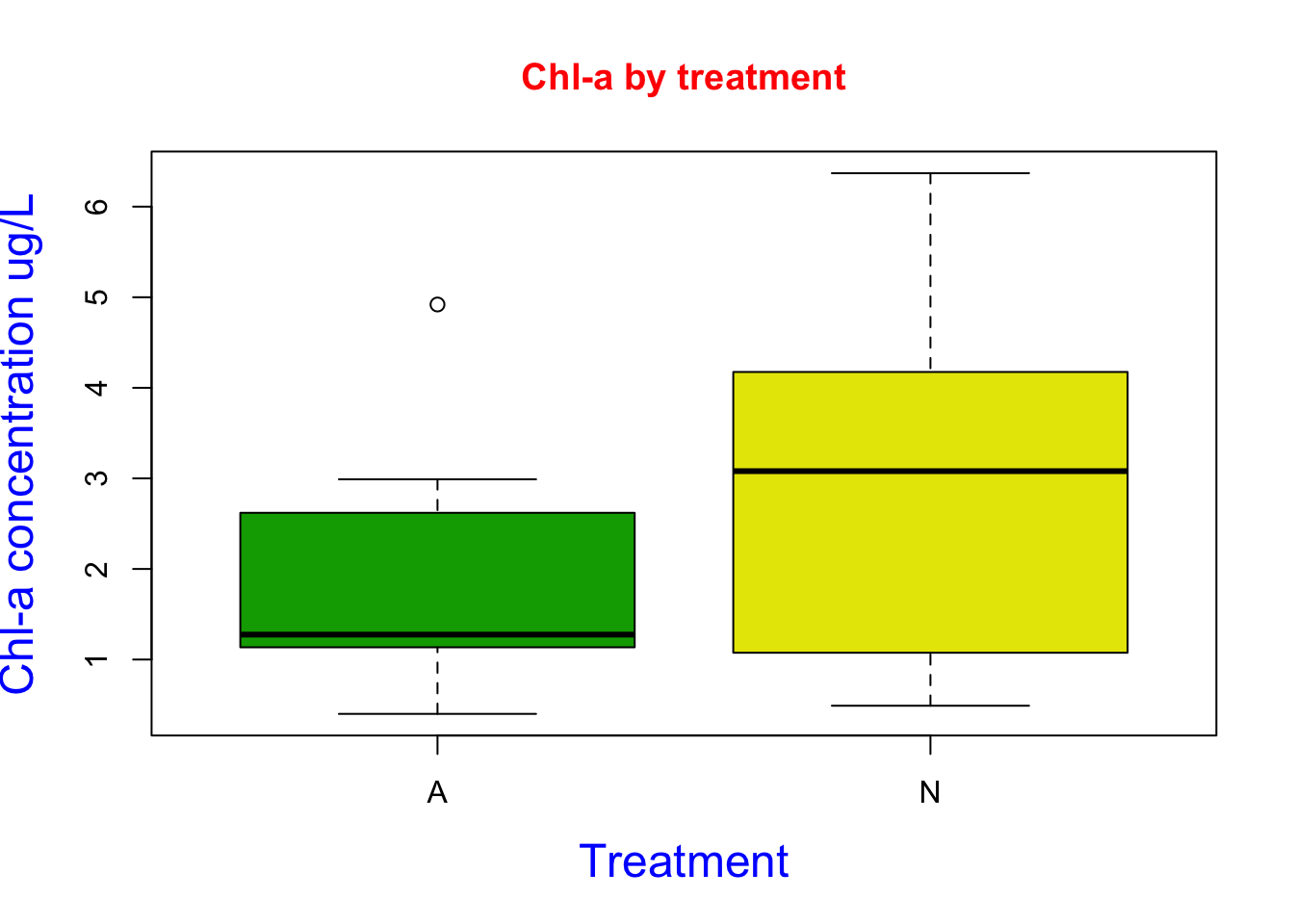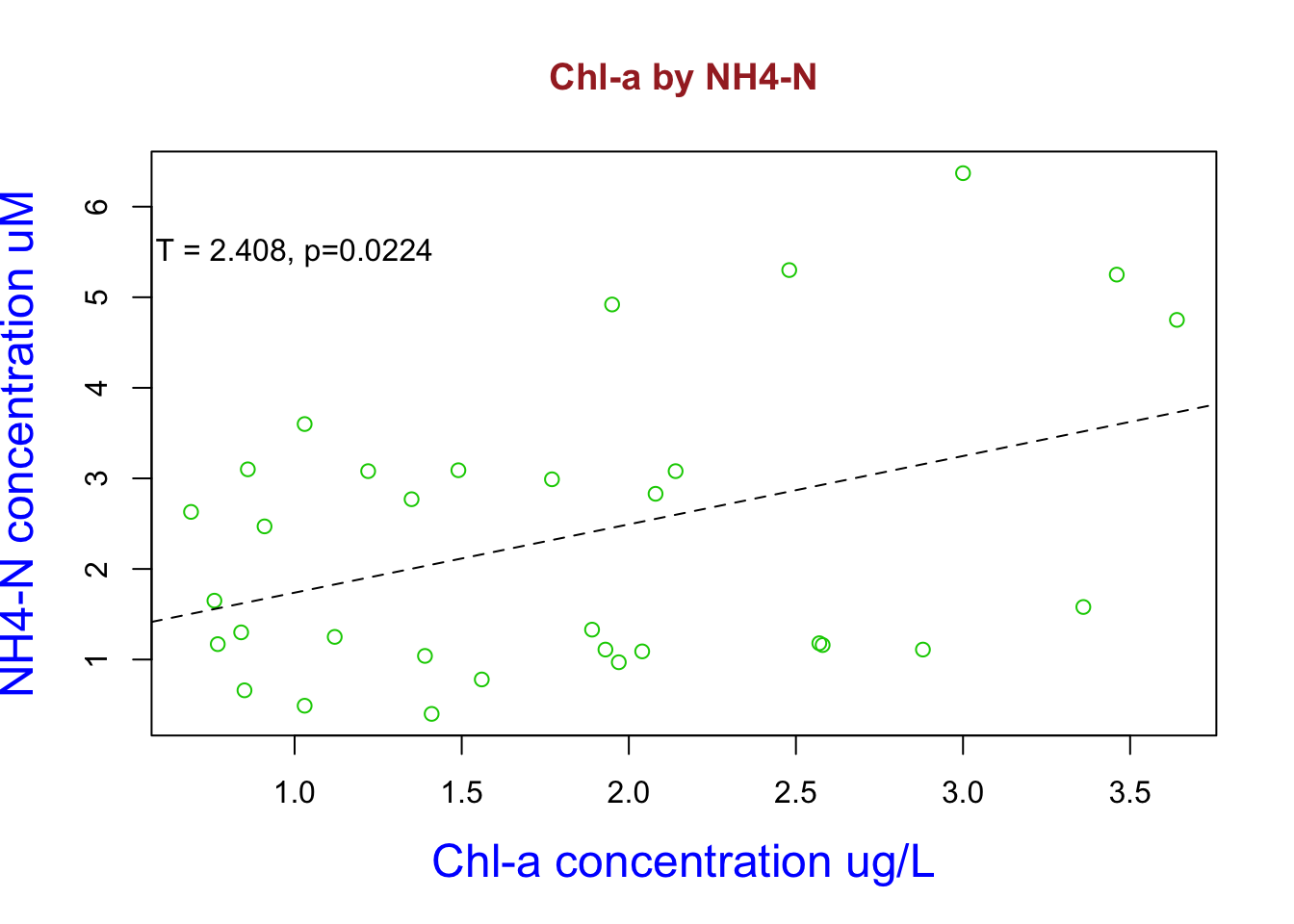# 3 Basic R Graphics

This is Chapter covers the basics of graphics and plots of R and RStudio.

## 3.1 Anatomy of a plot:

A figure consists of a plot region surrounded by margins,customized by function (par).

par(usr=c(x.lo, x.hi, y.lo, y.hi))#Setting plot region par(mfrow=c(1,3)) #Multiple plots

## 3.2 Margins

To change margins you can use the function (mar) or (mai)

mar=c(3,3,2,1) mai=c(0.5,0.5,0.25,0.5)

## 3.3 Axes and tick marks

Listed below are functions to access axes and tick marks 1) axes 2) bty 3) lab 4) las 5) tck 6) xaxs/yaxs

## 3.4 Common plot options (arguments)

1. cex: character size
2. col: color
3. pch: plotting symbols
4. lty, lwd: line type
5. log: lox scale axes
6. xlab, ylab: x and y labels
7. xlim, ylim: x and y variable range

## 3.5 My Data Example

Water samples were collected on 6/6/18, 9/24/18, 12/17/18, 1/26/19 and ran on an autoanalyzer to determine NO3-N, NH4-N, PO4, and Chl-a concentrations across 8 tanks identified by treatments (control "A" or nutrient enriched "N"). Moreover this data was used in my master's thesis.

library(readr)
Porewater_data <- read_csv("~/Desktop/R folder/Bookdown/Porewater_data.csv")
head(Porewater_data, n=3)
## # A tibble: 3 x 7
##    Tank Date   NO3 (uM) NH4 (uM) PO4 (uM) Conc of chl (ug/… Nutrient
##   <dbl> <chr>       <dbl>      <dbl>      <dbl>              <dbl> <chr>
## 1     1 6/6/18       2.07       1.35       3.08               2.77 A
## 2     2 6/6/18       4.68       2.48       2.45               5.3  N
## 3     3 6/6/18       3.05       1.77       4.62               2.99 A

Here I read in my porewater dataset and headed the first three lines.

boxplot(Porewater_data$Conc of chl (ug/L)~ Porewater_data$Nutrient,
col=terrain.colors(4),
main= "Chl-a by treatment", col.main="red",
xlab= "Treatment",
ylab= "Chl-a concentration ug/L",
col.lab="blue",
cex.lab=1.5)Here I created a boxplot using chl-a and treatment data. I used the col argument to designated the colors to terrain.color package. I added a title and axis labels using the main, xlab, and ylab arguments. I then changed the color of the labels using the col.main and col.lab argument. I then changed the font size of the labels using the cex.lab argument. Based on the figure we can see our treatment N increased chl-a concentrations.

plot(Porewater_data$Conc of chl (ug/L)~ Porewater_data$NH4 (uM),
col=3,
main= "Chl-a by NH4-N", col.main="Brown",
xlab= "Chl-a concentration ug/L",
ylab= "NH4-N concentration uM",
col.lab="blue",
cex.lab=1.5)

fit1 <- lm (Conc of chl (ug/L) ~ NH4 (uM), data = Porewater_data)
summary(fit1)
##
## Call:
## lm(formula = Conc of chl (ug/L) ~ NH4 (uM), data = Porewater_data)
##
## Residuals:
##     Min      1Q  Median      3Q     Max
## -2.0464 -1.3426 -0.1125  1.0466  3.1231
##
## Coefficients:
##             Estimate Std. Error t value Pr(>|t|)
## (Intercept)   0.9841     0.6175   1.594   0.1215
## NH4 (uM)    0.7543     0.3133   2.408   0.0224 *
## ---
## Signif. codes:  0 '***' 0.001 '**' 0.01 '*' 0.05 '.' 0.1 ' ' 1
##
## Residual standard error: 1.493 on 30 degrees of freedom
## Multiple R-squared:  0.1619, Adjusted R-squared:  0.134
## F-statistic: 5.797 on 1 and 30 DF,  p-value: 0.02241
abline(fit1, lty = "dashed")
text(x  =1.0, y = 5.5,labels="T = 2.408, p=0.0224")`** Here I used my dataset to look at the bivariate relationship between NH4-N and chl-a concentrations. Here I constructed a linear model and named it fit1. I then reviewed the statistics and it happens to be statistical significant. Increasing NH4 concentrations increases chl-a abundance. I add this the linear model regression line by using the abline argument and added the significant t and p values using the text argument**